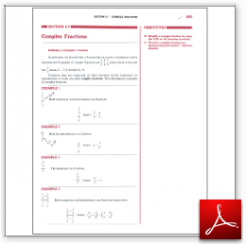Algebra Math Book - Introductory Algebra Thank you for your continual support.

 The power of mathematics rests in the logic of thinking.ID: Sec6-7
Description: Defining a Complex Fraction-Simplifying Complex Fractions-Using the LCD-Rewriting Complex Fractions
Price: 1.99

# Algebra Math book - Introductory Algebra - Chapter 6 - Section 7 - Complex Fractions

## Section 6.7 - Objectives

10.  Simplify a complex fraction by using the LCD of all fractions involved.

11.  Rewrite a complex fraction as a quotient using the division symbol and then simplify.

Navigate to
Previous Section or Next Section
or Chapter 6 Details or Other Chapters

This section of my algebra math book, Introductory Algebra, also includes in the download:

• Cover Sheet